Home Practice
For learners and parents For teachers and schools
Textbooks
Full catalogue
Pricing SupportLog in

We think you are located in United States. Is this correct?

# 4.4 Physical properties and structure

## 4.4 Physical properties and structure (ESCKP)

### Physical properties and intermolecular forces (ESCKQ)

Have the learners research the safety data for various compounds, especially those being used in the experiments in this section, as a way of linking the properties of organic molecules with their molecular structure.

The types of intermolecular forces that occur in a substance will affect its physical properties, such as its phase, melting point and boiling point. You should remember from the kinetic theory of matter (see Grade 10), that the phase of a substance is determined by how strong the forces are between its particles. The weaker the forces, the more likely the substance is to exist as a gas. This is because the particles are able to move far apart since they are not held together very strongly. If the forces are very strong, the particles are held closely together in a solid structure. Remember also that the temperature of a material affects the energy of its particles. The more energy the particles have, the more likely they are to be able to overcome the forces that are holding them together. This can cause a change in phase.

Figure 4.52 shows the three phases of water. Note that we are showing two-dimensional figures when in reality these are three-dimensional.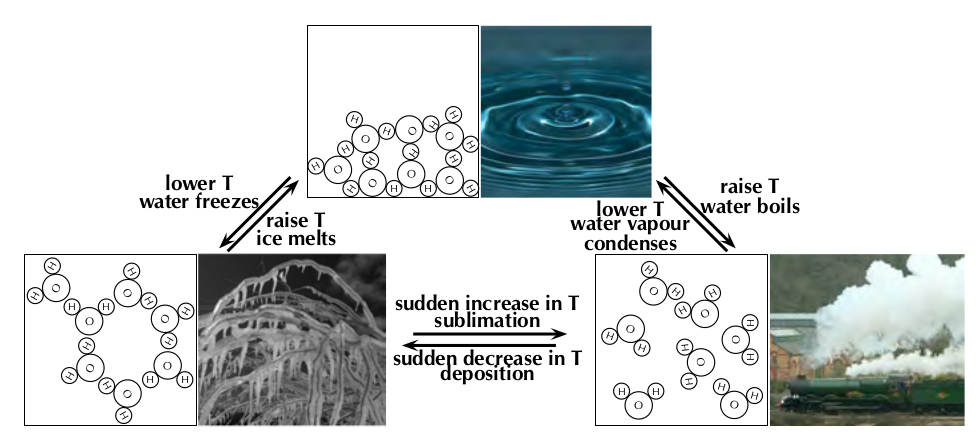Figure 4.52: Submicroscopic diagrams of the three phases of water. Photos by Iaszlo-photo, Fields of View and Capt' Gorgeous on Flickr.

#### The effects of intermolecular forces

The forces between molecules that bind them together are known as intermolecular forces. Intermolecular forces allow us to determine which substances are likely to dissolve in other substances, and what the melting and boiling points of substances are. Without intermolecular forces holding molecules together we would not exist.

Intermolecular forces

Intermolecular forces are forces that act between molecules.

Remember from Grade 11 that a dipole molecule is a molecule that has its charge unevenly distributed. One end of the molecule is slightly positive and the other is slightly negative. An overview of the different types of intermolecular forces that are discussed in this chapter are given below:

1. Dipole-dipole forces

When one dipole molecule comes into contact with another dipole molecule, the positive pole of the one molecule will be attracted to the negative pole of the other, and the molecules will be held together in this way.

One special case of this is hydrogen bonding:

Do not confuse hydrogen bonds with intramolecular covalent bonds. Hydrogen bonding is an example of a scientist naming something, believing it to be one thing when in fact it was another. In this case the strength of the hydrogen bonds misled scientists into thinking this was an intramolecular bond when it is really just a strong intermolecular force.

• Hydrogen bonds

As the name implies, this type of intermolecular bond involves a hydrogen atom. When a molecule contains a hydrogen atom covalently bonded to a small, highly electronegative atom (e.g. $$\text{O}$$, $$\text{N}$$ or $$\text{F}$$) this type of intermolecular force can occur. The highly electronegative atom on one molecule attracts the hydrogen atom on a nearby molecule (see Figure 4.53).

2. van der Waals forces

• Induced-dipole forces

Dipole-induced-dipole intermolecular forces are also sometimes called London forces or dispersion forces.

In non-polar molecules the electronic charge is usually evenly distributed but it is possible that at a particular moment in time, the electrons might not be evenly distributed (remember that the electrons are always moving in their orbitals). The molecule will have a temporary dipole. When this happens, molecules that are next to each other attract each other very weakly.

• Dipole-induced-dipole forces

These forces exist between dipoles and non-polar molecules. The dipole induces a dipole in the non-polar molecule leading to a weak, short lived force which holds the compounds together.

In this chapter we will focus on the effects of van der Waals forces and hydrogen bonding on the physical properties of organic molecules.

Viscosity

Viscosity is the resistance to flow of a liquid. Think how easy it is to pour water compared to syrup or honey. The water flows much faster than the syrup or honey.

Viscosity
Viscosity is a measure of how much a liquid resists flowing. i.e. The higher the viscosity, the more viscous a substance is.

You can see this if you take a cylinder filled with water and a cylinder filled with glycerol (propane-1,2,3-triol). Drop a small metal ball into each cylinder and note how easy it is for the ball to fall to the bottom (Figure 4.55). In the glycerol the ball falls slowly, while in the water it falls faster.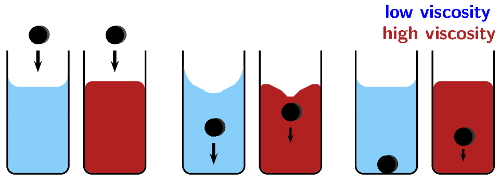Figure 4.55: The higher the viscosity (red) the slower the ball moves through the liquid.

As implied by the definition, substances with stronger intermolecular forces are more viscous than substances with weaker intermolecular forces. The stronger the intermolecular forces the more the substance will resist flowing. The greater the internal friction the more a substance will slow down an object moving through it.

If the glass sheet is tilted to either side during the resistance to flow activity the drops will run into each other and the activity will not work. The drops should reach the finish line in the order of: alcohol (least viscous), water, oil, syrup (most viscous).

## Resistance to flow

Take a sheet of glass at least $$\text{10}$$ by $$\text{15}$$ $$\text{cm}$$ in size. Using a water-proof marker draw a straight line across the width of the glass about $$\text{2}$$ $$\text{cm}$$ from each end.

Place the glass flat on top of two pencils, then carefully put a drop of water on one end of the line. Leave at least $$\text{2}$$ $$\text{cm}$$ space next to the water drop, and carefully place a drop of alcohol. Repeat this with a drop of oil and a drop of syrup.

Slowly and carefully remove the pencil from the end opposite the drops (make sure you don't tilt the glass to either side in the process).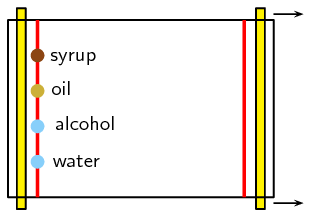• Which drop moves fastest and reaches the end line first?

• Which drop moves slowest?

The fastest moving substance has the least resistance to flow, and therefore has the least viscosity (is the least viscous). The slowest moving substance has the most resistance to flow, and therefore has the most viscosity (is the most viscous).

Density

Density
Density is a measure of the mass per unit of volume.

The solid phase is often the most dense phase (water is one noteworthy exception to this). This can be explained by the strong intermolecular forces found in a solid. These forces pull the molecules together, which results in more molecules in one unit of volume than in the liquid or gas phases. The more molecules in a unit volume the denser, and heavier that volume of the substance will be.

Density can be used to separate different liquids, with the more dense liquid settling to the bottom of the container, while the less dense liquid floats on top. If you throw a leaf into a river or pond the leaf will float. If you instead throw a rock (with the same surface area and volume) into the river or pond the rock will sink. This is due to the different densities of the two substances: rocks are more dense than water while leaves are less dense than water.

Melting and boiling points

Intermolecular forces affect the boiling and melting points of substances. Substances with weak intermolecular forces will have low melting and boiling points as less energy (heat) is needed to overcome these forces. Those with strong intermolecular forces will have high melting and boiling points as more energy (heat) is required to overcome these forces. When the temperature of a substance is raised beyond it's melting or boiling point the intermolecular forces are not weakened. Rather, the molecules have enough energy to overcome those forces.

An ether is a compound that contains two alkyl chains (e.g. methyl, ethyl) joined at an angle by an oxygen atom ($$-$$O$$-$$).

 Name Main intermolecular forces Molecular Mass ($$\text{g.mol^{-1}}$$) Melting point (℃) Boiling point (℃) Phase (at 25℃) ethane induced-dipole $$\text{30,06}$$ $$-\text{183}$$ $$-\text{89}$$ gas dimethyl ether dipole-dipole $$\text{46,06}$$ $$-\text{141}$$ $$-\text{24}$$ gas chloroethane dipole-dipole $$\text{64,5}$$ $$-\text{139}$$ $$\text{12,3}$$ gas pentane induced-dipole $$\text{72,12}$$ $$-\text{130}$$ $$\text{36}$$ liquid propan-1-ol hydrogen bonds $$\text{60,08}$$ $$-\text{126}$$ $$\text{97}$$ liquid ethanol hydrogen bonds $$\text{46,06}$$ $$-\text{114}$$ $$\text{78,4}$$ liquid butan-1-ol hydrogen bonds $$\text{74,1}$$ $$-\text{90}$$ $$\text{118}$$ liquid ethanoic acid hydrogen bonds $$\text{60,04}$$ $$\text{16,5}$$ $$\text{118,5}$$ liquid

Table 4.9: Relationship between intermolecular forces and melting point, boiling point and physical state.

As the intermolecular forces increase (from top to bottom in Table 4.9) the melting and boiling points increase. The stronger the intermolecular forces the more likely a substance is to be a liquid or a solid at room temperature.

## Worked example 29: Comparing physical properties

Given:

 Melting point (℃) Boiling point (℃) propane $$-\text{188}$$ $$-\text{42}$$ butanoic acid $$-\text{7,9}$$ $$\text{163,5}$$ bromoethane $$-\text{118}$$ $$\text{38,5}$$ diethyl ether $$-\text{116,3}$$ $$\text{34,6}$$

Fill in the table below:

 Name Main intermolecular forces Molecular formula Molecular mass ($$\text{g.mol^{-1}}$$) Phase (at 25 ℃) propane butanoic acid bromoethane diethyl ether

### Draw the structural representation of each molecule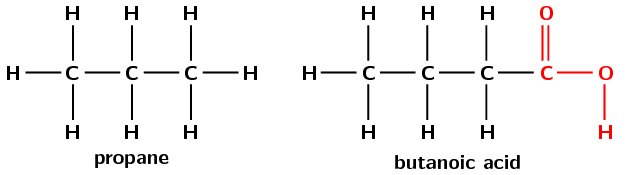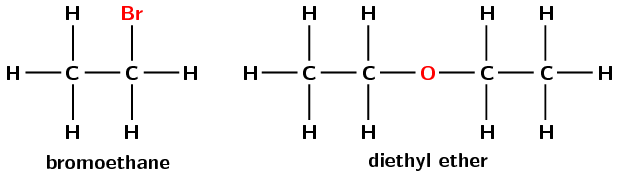### What are the intermolecular forces that each molecule will experience?

• propane has only single carbon-carbon bonds and no other functional group. It will therefore have induced-dipole forces only.

• butanoic acid has the carboxylic acid functional group. It can therefore form hydrogen bonds.

• bromoethane has the highly electronegative bromine atom. This means that it will form dipole-dipole interactions with neighbouring molecules.

• diethyl ether will have induced-dipole forces, however due to the flexible nature of the molecule is can also have dipole-induced-dipole (stronger van der Waals interactions) and dipole-dipole forces.

### Do these forces make sense with the melting and boiling points provided?

Propane has the lowest melting and boiling points and the weakest interactions. The next lowest melting and boiling points are for bromoethane and diethyl ether, which both have dipole-dipole interactions, the next strongest intermolecular forces. The highest melting and boiling points are for butanoic acid which has strong hydrogen bonds. Therefore these forces do make sense.

### Calculate the molecular mass of these molecules

• propane - $$\text{C}_{3}\text{H}_{8}$$, molecular mass = $$\text{44,08}$$ $$\text{g.mol^{-1}}$$

• butanoic acid - $$\text{C}_{4}\text{H}_{8}\text{O}_{2}$$, molecular mass = $$\text{88,08}$$ $$\text{g.mol^{-1}}$$

• bromoethane - $$\text{C}_{2}\text{H}_{5}\text{Br}$$, molecular mass = $$\text{108,95}$$ $$\text{g.mol^{-1}}$$

• diethyl ether - $$\text{C}_{4}\text{H}_{10}\text{O}$$, molecular mass = $$\text{74,10}$$ $$\text{g.mol^{-1}}$$

### What will the phase of each compound be at $$\text{25}$$ $$\text{℃}$$?

To determine the phase of a molecule at $$\text{25}$$ $$\text{℃}$$ look at the melting and boiling points:

• The melting and boiling points of propane are both below $$\text{25}$$ $$\text{℃}$$, therefore the molecule will be a gas at $$\text{25}$$ $$\text{℃}$$.

• The melting points of butanoic acid, bromoethane and dimethyl ether are below $$\text{25}$$ $$\text{℃}$$, however the boiling points of all three molecules are above $$\text{25}$$ $$\text{℃}$$. Therefore these molecules will be liquids at $$\text{25}$$ $$\text{℃}$$.

### Fill in the table

 Name Main intermolecular forces Molecular formula Molecular mass ($$\text{g.mol^{-1}}$$) Phase (at 25 ℃) propane induced-dipole $$\text{C}_{3}\text{H}_{8}$$ $$\text{44,08}$$ gas butanoic acid hydrogen bonds $$\text{C}_{4}\text{H}_{8}\text{O}_{2}$$ $$\text{88,08}$$ liquid bromoethane dipole-dipole $$\text{C}_{2}\text{H}_{5}\text{Br}$$ $$\text{108,95}$$ liquid diethyl ether dipole-dipole $$\text{C}_{4}\text{H}_{10}\text{O}$$ $$\text{74,10}$$ liquid

In the investigation of boiling and melting points experiment learners are required to work with ethyl methanoate. This can irritate your eyes, skin, nose and lungs. Please remind the learners to be careful and wear the appropriate safety equipment when handling all chemicals. The safety equipment includes gloves, safety glasses and protective clothing. Learners should also keep all open flames away from their experiment as they are handling flammable substances.

The boiling point of propanoic acid is approximately $$\text{141}$$ $$\text{℃}$$, which means that the cooking oil will be very hot by the time propanoic acid starts to boil. The learners should be reminded not to place their hands anywhere near the oil, and to allow it to cool before cleaning the apparatus.

This is a good opportunity to get the learners to research the hazard data sheets for butan-1-ol, propanoic acid and ethyl methanoate.

## Investigation of boiling and melting points

### Aim

To investigate the relationship between boiling points and intermolecular forces

### Apparatus

• butan-1-ol ($$\text{CH}_{2}(\text{OH})\text{CH}_{2}\text{CH}_{2}\text{CH}_{3}$$), propanoic acid ($$\text{CH}_{3}\text{CH}_{2}\text{COOH}$$) and ethyl methanoate ($$\text{HCOOCH}_{2}\text{CH}_{3}$$), cooking oil

• three test tubes, a beaker, a thermometer, a hot plate

### Method

Ethyl methanoate can irritate your eyes, skin, nose and lungs. Keep open flames away from your experiment and make sure you work in a well ventilated area.

1. Label the test tubes 1, 2 and 3. Place $$\text{20}$$ $$\text{ml}$$ of butan-1-ol into test tube 1, $$\text{20}$$ $$\text{ml}$$ of propanoic acid into test tube 2, and $$\text{20}$$ $$\text{ml}$$ of ethyl methanoate into test tube 3.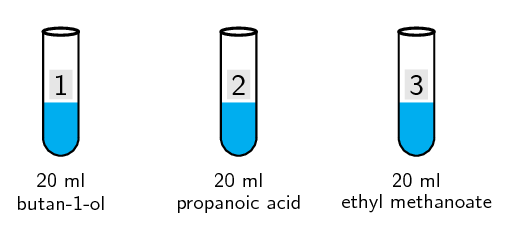2. Half-fill the beaker with cooking oil and place it on the hot plate.

3. Place the thermometer and the three test tubes in the beaker.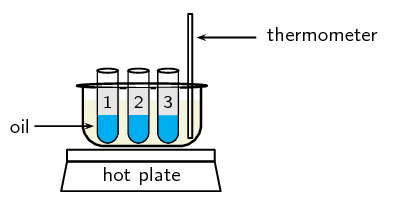4. Make a note of the temperature when each substance starts to boil.

### Results

Fill in the gaps in the table below. Do the values you obtained match those reported in literature?

 Compound ethyl methanoate butan-1-ol propanoic acid Molecular formula Molecular mass Main intermolecular forces Literature boiling point (℃) $$\text{54}$$ $$\text{118}$$ $$\text{141}$$ Experimental boiling point (℃)

Draw the structural representation of ethyl methanoate, butan-1-ol and propanoic acid.

### Discussion and conclusion

You should have found that the ethyl methanoate boiled first, then the butan-1-ol and then the propanoic acid. Ethyl methanoate has some dipole-dipole interactions, but cannot form a hydrogen bond. The alcohol (butan-1-ol) can form hydrogen bonds and so has a higher boiling point. This strong intermolecular force needs more energy to break and so the boiling point is higher. For propanoic acid hydrogen bonds form between the carbonyl group on one acid and the hydroxyl group on another. This means that each molecule of propanoic acid can be part of two hydrogen bonds (this is called dimerisation, see Figure 4.57) and so the boiling point is even higher for propanoic acid than for butan-1-ol.

Flammability and vapour pressure

Flammability is a measure of how easy it would be for a substance to catch alight and burn. The flash point of a substance is the lowest temperature that is likely to form a gaseous mixture you could set alight. If a liquid has a low enough flash point it is considered flammable (able to be ignited easily) while those with higher flash points are considered nonflammable. A substance that is classified as nonflammable can still be forced to burn, but it will not ignite easily.

When a substance is in the liquid or solid state there will be some molecules in the gas state. These molecules have enough energy to overcome the intermolecular forces holding the majority of the substance in the liquid or solid phase. These gas molecules exert a pressure on the liquid or solid (and the container) and that pressure is the vapour pressure of that compound. The weaker the intermolecular forces within a substance the higher the vapour pressure will be. Compounds with higher vapour pressures have lower flash points and are therefore more flammable.

Vapour pressure

The pressure exerted (at a specific temperature) on a solid or liquid compound by molecules of that compound that are in the gas phase.

 Name Main intermolecular forces Vapour pressure (kPa at 20℃) Flash point (℃) Flammability ethane induced-dipole $$\text{3 750}$$ $$-\text{135}$$ very high propane induced-dipole $$\text{843}$$ $$-\text{104}$$ very high dimethyl ether dipole-dipole $$\text{510}$$ $$\text{41}$$ very high butane induced-dipole $$\text{204}$$ $$-\text{60}$$ very high chloroethane dipole-dipole $$\text{132,4}$$ $$-\text{50}$$ very high pentane induced-dipole $$\text{57,9}$$ $$-\text{49}$$ very high propanone dipole-dipole $$\text{24,6}$$ $$-\text{17}$$ high ethanol hydrogen bonds $$\text{5,8}$$ $$\text{17}$$ high water hydrogen bonds $$\text{2,3}$$ - very low propan-1-ol hydrogen bonds $$\text{2}$$ $$\text{22}$$ high ethanoic acid hydrogen bonds $$\text{1,6}$$ $$\text{40}$$ moderate butan-1-ol hydrogen bonds $$\text{0,6}$$ $$\text{35}$$ high

Table 4.10: Relationship between intermolecular forces and the flammability of a substance.

As the intermolecular forces increase (from top to bottom in Table 4.10) you can see a decrease in the vapour pressure. This corresponds with an increase in the flash point temperature and a decrease in the flammability of the substance. Figure 4.58 shows a few examples.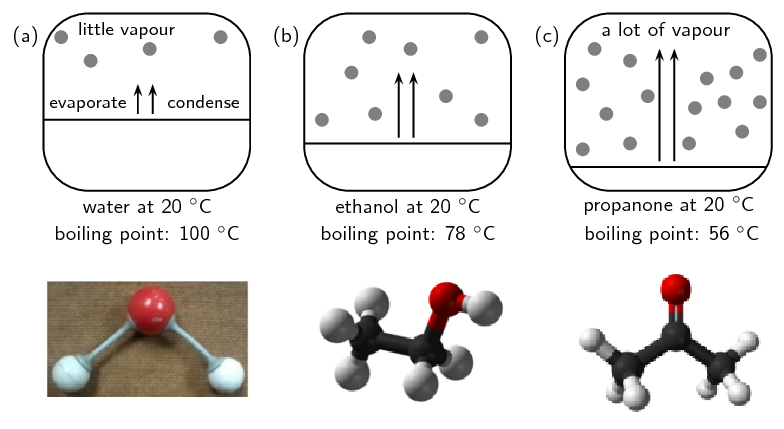Figure 4.58: The vapour pressure of (a) water, (b) ethanol and (c) propanone at $$\text{20}$$ $$\text{℃}$$. Images by Duncan Watson.

## Types of intermolecular forces

Textbook Exercise 4.19

Use your knowledge of different types of intermolecular forces to explain the following statements:

The boiling point of hex-1-ene is much lower than the boiling point of propanoic acid.

Propanoic acid has hydrogen bonds which are much stronger than the induced-dipole forces in hex-1-ene. In order for a liquid to boil the intermolecular forces must be broken. The stronger the intermolecular forces, the more energy it will take to overcome these forces. As a result the boiling point will be higher for propanoic acid than for hex-1-ene.

Water evaporates more slowly than propanone.

Water has strong intermolecular forces (hydrogen bonds) while propanone only has weaker dipole-dipole forces. Substances with stronger intermolecular forces take longer to evaporate than substances with weaker intermolecular forces.

 IUPAC name Boiling point (℃) A ethane $$-\text{89,0}$$ B ethanol $$\text{78,4}$$ C ethanoic acid $$\text{118,5}$$

Which of the compounds listed in the table are gases at room temperature?

ethane

Name the main type of intermolecular forces for A, B and C.

A - induced-dipole forces

B - hydrogen bonding

C - hydrogen bonding

Account for the difference in boiling point between A and B.

A has only induced-dipole forces (weak van der Waals forces). B has hydrogen bonding. Since hydrogen bonding is a stronger intermolecular force than van der Waals forces, more energy is required to separate the molecules of ethanol than the molecules of ethane. Thus ethanol has a higher boiling point than ethane.

Account for the difference in boiling point between B and C.

Both B and C undergo hydrogen bonding. However the hydrogen bonding in C (carbonyl and hydroxyl group) is stronger than that of B (hydroxyl group). This is because C forms a hydrogen bonding dimer (see Figure 4.57), while B forms only single hydrogen bonds. Thus C has a higher boiling point than B.

Draw the structural representations of A, B and C.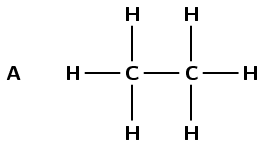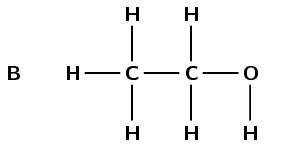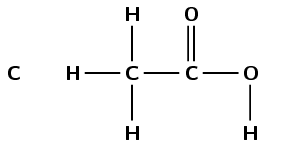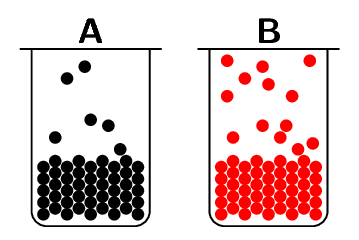Which container (A or B) has the compound with higher vapour pressure in it? Explain your answer.

B. There are more molecules of B in the vapour phase than there are of A. More molecules in the vapour phase means a higher vapour pressure.

Draw the condensed structural formula for each of these compounds:

propanoic acid

$$\text{CH}_{3}\text{CH}_{2}\text{COOH}$$

butan-1-ol

$$\text{CH}_{2}(\text{OH})\text{CH}_{2}\text{CH}_{2}\text{CH}_{3}$$

Propanoic acid has a vapour pressure of $$\text{0,32}$$ $$\text{kPa}$$ at $$\text{20}$$ $$\text{℃}$$

Butan-1-ol has a vapour pressure of $$\text{0,64}$$ $$\text{kPa}$$ at $$\text{20}$$ $$\text{℃}$$

Explain the difference in vapour pressure.

Both compounds have the same molecular mass, but propanoic acid has two sites for hydrogen bonding (can form a hydrogen bonding dimer, see Figure 4.57) while butan-1-ol has only one. So butan-1-ol has weaker intermolecular forces and will go into the vapour phase easier than propanoic acid. Butan-1-ol therefore has a higher vapour pressure than propanoic acid.

### Physical properties and functional groups (ESCKR)

Compounds that contain very similar atoms can have very different properties depending on how those atoms are arranged. This is especially true when they have different functional groups. Table 4.11 shows some properties of different functional groups.

Vanilla has a sweet smell. The smell of ethene and dimethyl ether cling in your nose though, and the smell of ether can even stay on your skin up to $$\text{24}$$ hours.

 Functional group Typical Smell Example Formula Melting point (℃) Boiling point (℃) Phase (at 25℃) alkane odourless ethane $$\text{C}_{2}\text{H}_{6}$$ $$-\text{183}$$ $$-\text{89}$$ gas alkene sweet/musky ethene $$\text{C}_{2}\text{H}_{4}$$ $$-\text{169,2}$$ $$-\text{103,7}$$ gas ether (-O-) sweet dimethyl ether $$\text{C}_{2}\text{H}_{6}\text{O}$$ $$-\text{141}$$ $$-\text{24}$$ gas haloalkane almost odourless chloro ethane $$\text{C}_{2}\text{H}_{5}\text{Cl}$$ $$-\text{139}$$ $$\text{12,3}$$ gas aldehyde pungent fruity ethanal $$\text{C}_{2}\text{H}_{4}\text{O}$$ $$-\text{123,4}$$ $$\text{20,2}$$ gas alcohol sharp ethanol $$\text{C}_{2}\text{H}_{6}\text{O}$$ $$-\text{114}$$ $$\text{78,4}$$ liquid ester often fruity methyl methanoate $$\text{C}_{2}\text{H}_{4}\text{O}_{2}$$ $$-\text{100}$$ $$\text{32}$$ liquid alkyne odourless ethyne $$\text{C}_{2}\text{H}_{2}$$ $$-\text{80,8}$$ $$-\text{84}$$ gas carboxylic acid vinegar rancid butter ethanoic acid $$\text{C}_{2}\text{H}_{4}\text{O}_{2}$$ $$\text{16,5}$$ $$\text{118,5}$$ liquid

Table 4.11: Some properties of compounds with different functional groups.

Listed in the table are the common smells and other physical properties found for common functional groups. Only one representative example from each homologous series is provided. This does not mean that all compounds in that series have exactly the same properties. For example, short-chain and long-chain alkanes are generally odourless, while those with moderate chain length (approximately $$\text{6}$$ - $$\text{12}$$ carbon atoms) smell like petrol (Table 4.15).

Some specific properties of a few functional groups will now be discussed in more detail.

#### Physical properties of the alcohols

In humans, ethanol reduces the secretion of a hormone called antidiuretic hormone (ADH). The role of ADH is to control the amount of water that the body retains. When this hormone is not secreted in the right quantities, it can cause dehydration because too much water is lost from the body in the urine (ethanol is a diuretic). This is why people who drink too much alcohol can become dehydrated, and experience symptoms such as headaches, dry mouth, and lethargy. Part of the reason for the headaches is that dehydration causes the brain to shrink away from the skull slightly.

Ethane has a relatively low solubility in water.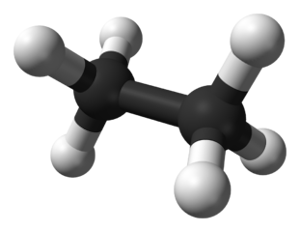Because hydroxyl ($$-\text{OH}$$) groups can hydrogen bond, all three pentanol molecules have a greater solubility in water than ethane.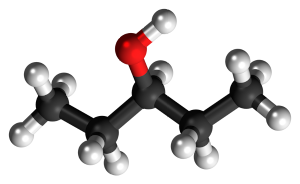Ethanol is completely soluble in water in any amount. This is because it contains a hydroxyl group and has a much shorter non-polar chain length than pentanol.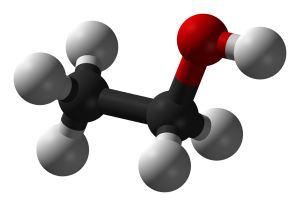pentanol solubility ($$\text{g.dm^{-3}}$$) ethane $$\text{0,057}$$ 1-pentanol $$\text{22}$$ 2-pentanol $$\text{45}$$ 3-pentanol $$\text{59}$$ ethanol soluble

The hydroxyl group ($$-\text{OH}$$) affects the solubility of the alcohols.

Solubility
Solubility is a measure of the ability of a substance (solid, liquid or gas) to dissolve in another substance. The amount of the substance than can dissolve is the measure of its solubility.

The hydroxyl group generally makes the alcohol molecule polar and therefore more likely to be soluble in water. However, the carbon chain resists solubility, so there are two opposing trends in the alcohols. Alcohols with shorter carbon chains are usually more soluble in water than those with longer carbon chains.

Alcohols tend to have higher boiling points than the hydrocarbons because of the strong hydrogen bond between hydrogen atoms of one hydroxyl group and the oxygen atom of another hydroxyl group.

#### Physical properties of haloalkanes

There can be more than one halogen substituted for a hydrogen atom on one haloalkane. The more halogens are substituted the less volatile the haloalkane becomes. Take for example the haloalkane series shown in Figure 4.59.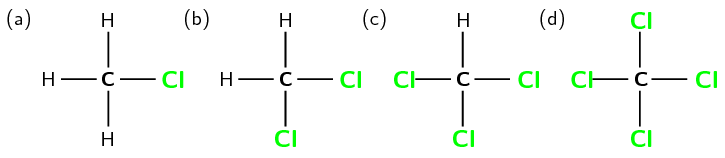Figure 4.59: A series of haloalkanes with increasing numbers of chlorine atoms: (a) chloromethane, (b) dichloromethane, (c) trichloromethane and (d) tetracholormethane.
Volatility
The tendency of molecules at the surface of a compound to enter the gas phase. The more volatile a compound the more likely this is.

For every extra chlorine atom on the original methane molecule the volatility of the compound decreases. This can be seen by the increase in both the melting and boiling point (Table 4.12) as one goes from chloromethane through to tetrachloromethane. The more halogen atoms in the compound the stronger the intermolecular forces are, which leads to higher melting and boiling points.

 Common Name Number Cl atoms Melting point (℃) Boiling point (℃) chloromethane $$\text{1}$$ $$-\text{97,4}$$ $$-\text{24,2}$$ dichloromethane $$\text{2}$$ $$-\text{96,7}$$ $$\text{39,6}$$ trichloromethane $$\text{3}$$ $$-\text{63,5}$$ $$\text{61,2}$$ tetrachloromethane $$\text{4}$$ $$-\text{22,9}$$ $$\text{76,7}$$

Table 4.12: Melting and boiling points of haloalkanes with increasing numbers of chlorine atoms.

#### Properties of carbonyl compounds

Carboxylic acids are weak acids, in other words they only dissociate partially. Why does the carboxyl group have acidic properties? In the carboxyl group, the hydrogen tends to separate itself (dissociate) from the oxygen atom. In other words, the carboxyl group becomes a source of positively-charged hydrogen ions ($$\text{H}^{+}$$). This is shown in Figure 4.60.

The carboxylic acid functional group is soluble in water. However, as the number of carbon atoms in the attached carbon chain increases the solubility decreases. This is discussed in greater detail in the next section.

Remember that carboxylic acids form hydrogen bonding dimers (the formation is called dimerisation) as shown in Figure 4.61.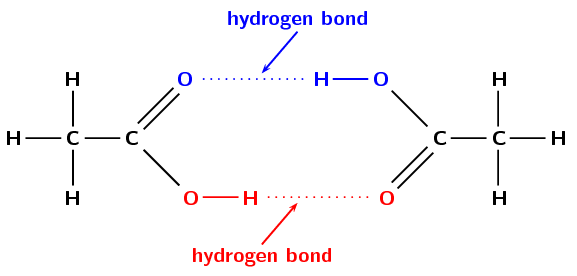Figure 4.61: Ethanoic acid forming a carboxylic acid hydrogen bonding dimer.

The ability of a molecule to hydrogen bond leads to increased melting and boiling points when compared to a similar molecule that is unable to hydrogen bond. Similarly, the ability of a molecule to form a hydrogen bonding dimer leads to increased melting and boiling points when compared to similar molecules that can only form one hydrogen bond (Table 4.13).

 Molecule Hydrogen bonds per molecule Melting point (℃) Boiling point (℃) ethane 0 -183 -89 ethanol 1 -114 $$\text{78,4}$$ ethanoic acid 2 $$\text{16,5}$$ $$\text{118,5}$$

Table 4.13: The melting and boiling points of similar organic compounds that can form different numbers of hydrogen bonds.

#### Physical properties of ketones

Hydrogen bonds are stronger than the van der Waals forces found in ketones. Therefore compounds with functional groups that can form hydrogen bonds are more likely to be soluble in water. This applies to aldehydes as well as to ketones.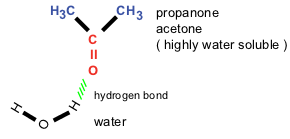Figure 4.62: The hydrogen bond between water and a ketone (propanone).

## Physical properties and functional groups

Textbook Exercise 4.20

Refer to the data table below which shows the melting point and boiling point for a number of organic compounds with different functional groups.

 Formula Name Melting point (℃) Boiling point (℃) $$\text{C}_{4}\text{H}_{10}$$ butane $$-\text{137}$$ $$\text{0}$$ $$\text{C}_{4}\text{H}_{8}$$ but-1-ene $$-\text{185}$$ $$-\text{6,5}$$ $$\text{C}_{4}\text{H}_{10}\text{O}$$ butan-1-ol $$-\text{90}$$ $$\text{118}$$ $$\text{C}_{4}\text{H}_{8}\text{O}_{2}$$ butanoic acid $$-\text{7,9}$$ $$\text{163,5}$$ $$\text{C}_{5}\text{H}_{12}$$ pentane $$-\text{130}$$ $$\text{36}$$ $$\text{C}_{5}\text{H}_{10}$$ pent-1-ene $$-\text{165,2}$$ $$\text{30}$$ $$\text{C}_{5}\text{H}_{12}\text{O}$$ pentan-1-ol $$-\text{78}$$ $$\text{138}$$ $$\text{C}_{5}\text{H}_{10}\text{O}_{2}$$ pentanoic acid $$-\text{34,5}$$ $$\text{186,5}$$

At room temperature (approx. $$\text{25}$$$$\text{°C}$$), which of the organic compounds in the table are:

gases

butane, but-1-ene

liquids

All except butane and but-1-ene

Look at an alkane, alkene, alcohol and carboxylic acid with the same number of carbon atoms:

How do their melting and boiling points compare?

The carboxylic acids have the highest melting and boiling points, then the alcohols, then the alkanes and the alkenes have the lowest melting and boiling points.

Looking at those compounds with four carbon atoms:

Butanoic acid has the highest boiling (and melting) point, the next highest boiling (and melting) point is butan-1-ol, then butane (the alkane) followed by but-1-ene (the alkene).

Looking at those compounds with five carbon atoms:

Pentanoic acid has the highest boiling (and melting) point, the next highest boiling (and melting) point is pentan-1-ol, then pentane (the alkane) followed by pent-1-ene (the alkene).

Explain why their melting points and boiling points are different?

The carboxylic acids have the strongest intermolecular forces due to their ability to form two hydrogen bonds (dimerisation). The alcohols can only form one hydrogen bond leading to lower melting and boiling points. The alkenes and alkanes cannot form hydrogen bonds. However, the alkenes are more reactive than the alkanes due to the double bond (unsaturated) and so the melting and boiling points of an alkene are lower than those of an alkane with the same number of carbon atoms.

### Physical properties and chain length (ESCKS)

Remember that the alkanes are a group of organic compounds that contain carbon and hydrogen atoms bonded together. The carbon atoms link together to form chains of varying lengths. We have already mentioned that the alkanes are relatively unreactive because of their stable C-C and C-H bonds. The boiling points and melting points of these molecules are determined by their molecular structure and their surface area.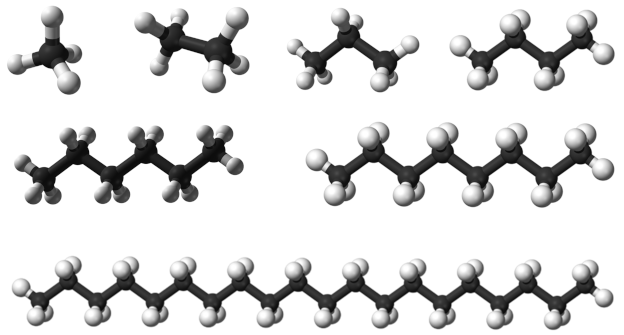Figure 4.63: Some alkanes, from top left to bottom right: methane, ethane, propane, butane, hexane, octane and icosane.

The more carbon atoms there are in an alkane, the greater the surface area available for intermolecular interactions.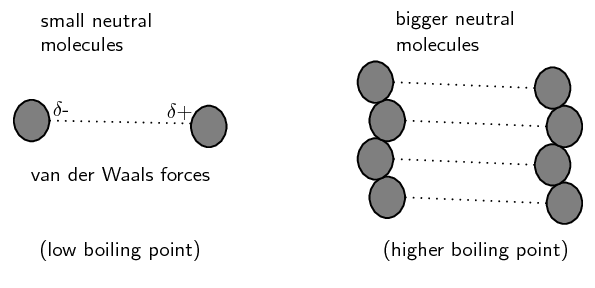Figure 4.64: van der Waals intermolecular forces increase as chain length increases. Image by Duncan Watson.

This increase in intermolecular attractions leads to higher melting and boiling points. This is shown in Table 4.14.

 Formula Name Molecular mass ($$\text{g.mol^{-1}}$$) Melting point (℃) Boiling point (℃) Phase (at 25℃) $$\text{CH}_{4}$$ methane $$\text{16,04}$$ $$-\text{182}$$ $$-\text{162}$$ gas $$\text{C}_{2}\text{H}_{6}$$ ethane $$\text{30,06}$$ $$-\text{183}$$ $$-\text{89}$$ gas $$\text{C}_{3}\text{H}_{8}$$ propane $$\text{44,08}$$ $$-\text{188}$$ $$-\text{42}$$ gas $$\text{C}_{4}\text{H}_{10}$$ butane $$\text{58,1}$$ $$-\text{137}$$ $$\text{0}$$ gas $$\text{C}_{6}\text{H}_{14}$$ hexane $$\text{86,14}$$ $$-\text{95}$$ $$\text{68,5}$$ liquid $$\text{C}_{8}\text{H}_{18}$$ octane $$\text{114,18}$$ $$-\text{57}$$ $$\text{125,5}$$ liquid $$\text{C}_{20}\text{H}_{42}$$ icosane $$\text{282,42}$$ $$\text{37}$$ $$\text{343}$$ solid

Table 4.14: The physical properties of some alkanes.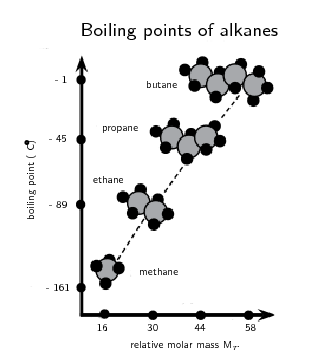Figure 4.65: As the molar mass of an alkane increases so does the boiling point. Image by Duncan Watson.

Notice that when the molecular mass of the alkanes is low (i.e. there are few carbon atoms), the organic compounds are gases because the intermolecular forces are weak. As the number of carbon atoms and the molecular mass increases, the compounds are more likely to be liquids or solids because the intermolecular forces are stronger.

The larger a molecule is, the stronger the intermolecular forces are between the molecules. This is one of the reasons why methane ($$\text{CH}_{4}$$) is a gas at room temperature while hexane ($$\text{C}_{6}\text{H}_{14}$$) is a liquid and icosane ($$\text{C}_{20}\text{H}_{42}$$) is a solid.

Be careful when comparing molecules with different types of intermolecular forces. For example, small molecule with hydrogen bonding can have stronger intermolecular forces than a large molecule with only van der Waals forces.

Table 4.15 shows some other properties of the alkanes that also vary with chain length.

 Name Density ($$\text{g.dm^{-3}}$$) Flash point (℃) Smell Phase (at 25℃) methane $$\text{0,66}$$ $$-\text{188}$$ odourless gas ethane $$\text{1,28}$$ $$-\text{135}$$ odourless gas propane $$\text{2,01}$$ $$-\text{104}$$ odourless gas butane $$\text{2,48}$$ $$-\text{60}$$ like petrol gas hexane $$\text{650}$$ $$-\text{26}$$ like petrol liquid octane $$\text{700}$$ $$\text{13}$$ like petrol liquid icosane $$\text{790}$$ $$>\text{113}$$ odourless solid

Table 4.15: Properties of some of the alkanes.

Density increases with increasing molecular size. Note that compounds that are gaseous are much less dense than compounds that are liquid or solid.

Remember that the flash point of a volatile molecule is the lowest temperature at which that molecule can form a vapour mixture with air and be ignited. The flash point increases with increasing chain length meaning that the longer chains are less flammable (Table 4.10) although they can still be ignited.

It is partly the stronger intermolecular forces that explain why petrol (mainly octane ($$\text{C}_{8}\text{H}_{18}$$)) is a liquid, while candle wax ($$\text{C}_{23}\text{H}_{48}$$) is a solid. If these intermolecular forces did not increase with increasing molecular size we would not be able to put liquid fuel into our cars or use solid candles.

The change in physical properties due to chain length does not only apply to the hydrocarbons. The solubility of ketones in water decreases as the chain length increases. The longer the chain length, the stronger the intermolecular interactions between the ketone molecules. This means that more energy is required to overcome those interactions and the molecule is therefore less soluble in water. Long chains can also fold around the polar carbonyl group and stop water molecules from bonding with it.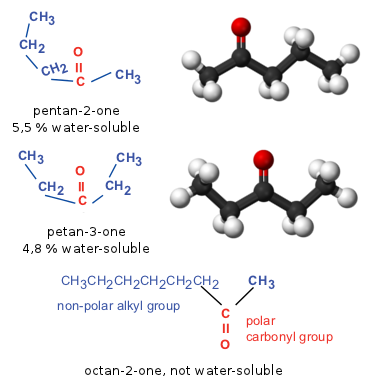Figure 4.66: The water solubility of a ketone decreases as the chain length increases.

This same idea can be applied to all the compounds with water-soluble functional groups. The longer the chain becomes, the less soluble the compound is in water.

## Physical properties and chain length

Textbook Exercise 4.21

Refer to the table below which gives information about a number of carboxylic acids, and then answer the questions that follow.

 Condensed formula Common name Source IUPAC name Melting point (℃) Boiling point (℃) formic acid ants methanoic acid $$\text{8,4}$$ $$\text{101}$$ $$\text{CH}_{3}\text{COOH}$$ vinegar ethanoic acid $$\text{16,5}$$ $$\text{118,5}$$ propionic acid milk propanoic acid $$-\text{20,8}$$ $$\text{141}$$ $$\text{CH}_{3}(\text{CH}_{2})_{2}\text{COOH}$$ butyric acid butter $$-\text{7,9}$$ $$\text{163,5}$$ valeric acid valerian root pentanoic acid $$-\text{34,5}$$ $$\text{186,5}$$ $$\text{CH}_{3}(\text{CH}_{2})_{4}\text{COOH}$$ caproic acid goat skin $$-\text{3,4}$$ $$\text{205,8}$$ enanthic acid vines heptanoic acid $$-\text{7,5}$$ $$\text{223}$$ $$\text{CH}_{3}(\text{CH}_{2})_{6}\text{COOH}$$ caprylic acid goat milk $$\text{16,7}$$ $$\text{239,7}$$

Fill in the missing spaces in the table by writing the formula, common name or IUPAC name.

 Condensed formula Common name Source IUPAC name Melting point (℃) Boiling point (℃) $$\text{HCOOH}$$ formic acid ants methanoic acid $$\text{8,4}$$ $$\text{101}$$ $$\text{CH}_{3}\text{COOH}$$ acetic acid vinegar ethanoic acid $$\text{16,5}$$ $$\text{118,5}$$ $$\text{CH}_{3}\text{CH}_{2}\text{COOH}$$ propionic acid milk propanoic acid $$-\text{20,8}$$ $$\text{141}$$ $$\text{CH}_{3}(\text{CH}_{2})_{2}\text{COOH}$$ butyric acid butter butanoic acid $$-\text{7,9}$$ $$\text{163,5}$$ $$\text{CH}_{3}(\text{CH}_{2})_{3}\text{COOH}$$ valeric acid valerian root pentanoic acid $$-\text{34,5}$$ $$\text{186,5}$$ $$\text{CH}_{3}(\text{CH}_{2})_{4}\text{COOH}$$ caproic acid goat skin hexanoic acid $$-\text{3,4}$$ $$\text{205,8}$$ $$\text{CH}_{3}(\text{CH}_{2})_{5}\text{COOH}$$ enanthic acid vines heptanoic acid $$-\text{7,5}$$ $$\text{223}$$ $$\text{CH}_{3}(\text{CH}_{2})_{6}\text{COOH}$$ caprylic acid goat milk octanoic acid $$\text{16,7}$$ $$\text{239,7}$$

Draw the structural representation of butyric acid.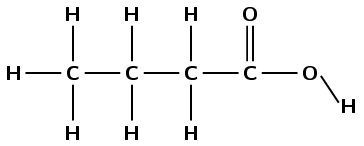Give the molecular formula for caprylic acid.

$$\text{C}_{8}\text{H}_{16}\text{O}_{2}$$

Draw a graph to show the relationship between molecular mass (on the x-axis) and boiling point (on the y-axis)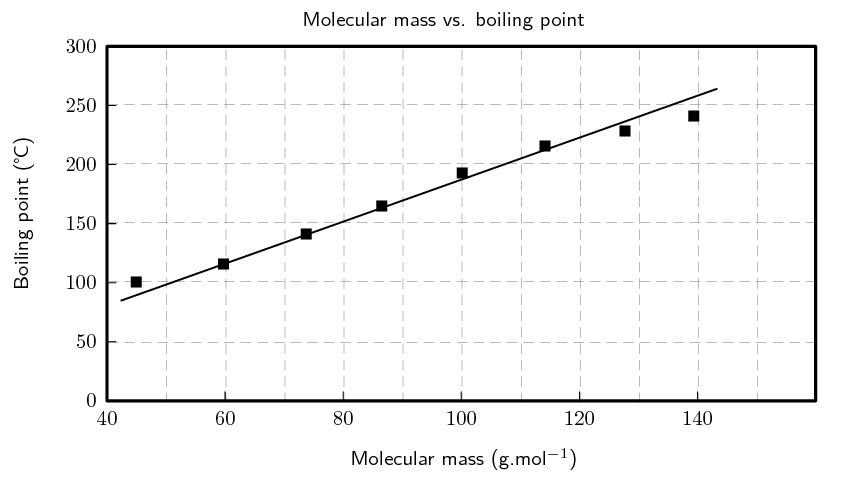Describe the trend you see.

As the molecular mass increases, so does the boiling point OR boiling point increases with an increase in molecular mass (something along these lines).

Suggest a reason for this trend.

Longer chains allow for greater van der Waals interactions leading to higher boiling points OR an increase in the surface area of the molecules leads to greater intermolecular forces which leads to increasing boiling points OR the forces holding the molecules in the solid phase are increasing and so the boiling point increases, this is because the compounds with larger molecular mass have longer chains (something along these lines).

Refer to the data table below which shows the melting point and boiling point for a number of organic compounds with different functional groups.

 Formula Name Melting point (℃) Boiling point (℃) $$\text{C}_{4}\text{H}_{10}$$ Butane $$-\text{137}$$ $$\text{0}$$ $$\text{C}_{5}\text{H}_{12}$$ Pentane $$-\text{130}$$ $$\text{36}$$ $$\text{C}_{6}\text{H}_{14}$$ Hexane $$-\text{95}$$ $$\text{68,5}$$ $$\text{C}_{4}\text{H}_{8}$$ But-1-ene $$-\text{185}$$ $$-\text{6,5}$$ $$\text{C}_{5}\text{H}_{10}$$ Pent-1-ene $$-\text{165,2}$$ $$\text{30}$$ $$\text{C}_{6}\text{H}_{12}$$ Hex-1-ene $$-\text{140}$$ $$\text{63}$$

At room temperature (approx. 25 °C), which of the organic compounds in the table are:

gases

butane, butene

liquids

pentane, pentene, hexane, hexene

In the alkanes:

Describe what happens to the melting point and boiling point as the number of carbon atoms in the compound increases.

The melting and boiling points increase as the number of carbon atoms increase.

Explain why this is the case.

As the number of carbon atoms increases, so does the surface area of the molecule. This leads to greater intermolecular forces, which are responsible for the increase in melting and boiling points.

Fill in the table below. Under boiling point put 1 for the compound with the lowest boiling point, 2 for the next lowest boiling point until you get to 6 for the compound with the highest boiling point. Do not use specific boiling point values, but rather use your knowledge of intermolecular forces.

 Compound Condensed Structure Boiling point propane $$\text{CH}_{3}\text{CH}_{2}\text{CH}_{3}$$ hexane $$\text{CH}_{3}\text{CH}_{2}\text{CH}_{2}\text{CH}_{2}\text{CH}_{2}\text{CH}_{3}$$ methane $$\text{CH}_{4}$$ e.g. 1 octane $$\text{CH}_{3}\text{CH}_{2}\text{CH}_{2}\text{CH}_{2}\text{CH}_{2}\text{CH}_{2}\text{CH}_{2}\text{CH}_{3}$$ butane $$\text{CH}_{3}\text{CH}_{2}\text{CH}_{2}\text{CH}_{3}$$ ethane $$\text{CH}_{3}\text{CH}_{3}$$
 Compound Condensed Structure Boiling point propane $$\text{CH}_{3}\text{CH}_{2}\text{CH}_{3}$$ 3 hexane $$\text{CH}_{3}\text{CH}_{2}\text{CH}_{2}\text{CH}_{2}\text{CH}_{2}\text{CH}_{3}$$ 5 methane $$\text{CH}_{4}$$ 1 octane $$\text{CH}_{3}\text{CH}_{2}\text{CH}_{2}\text{CH}_{2}\text{CH}_{2}\text{CH}_{2}\text{CH}_{2}\text{CH}_{3}$$ 6 butane $$\text{CH}_{3}\text{CH}_{2}\text{CH}_{2}\text{CH}_{3}$$ 4 ethane $$\text{CH}_{3}\text{CH}_{3}$$ 2

Draw the structural representations for each of the following compounds and answer the question that follows.

but-2-yne

The prefix but- tells us that there are four carbon atoms in the longest chain. The suffix -2-yne tells us that there is a triple bond between the second and third carbon atoms.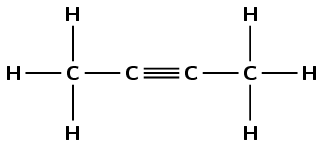hex-2-yne

The prefix hex- tells us that there are six carbon atoms in the longest chain. The suffix -2-yne tells us that there is a triple bond between the second and third carbon atoms.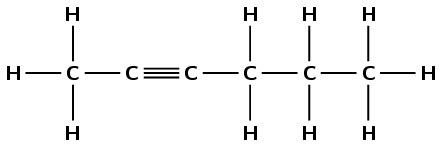pent-2-yne

The prefix pent- tells us that there are five carbon atoms in the longest chain. The suffix -2-yne tells us that there is triple bond between the second and third carbon atoms.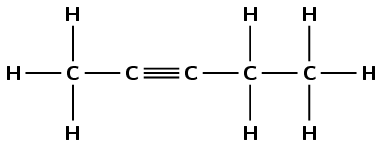Which of these compounds will have the highest viscosity?

hex-2-yne - All three compounds are alkynes. There is an increase in chain length with but-2-yne having the shortest chain and hex-2-yne the longest. The van der Waals forces will be stronger in the longest chain compound, hex-2-yne, because it has more sites for the van der Waals forces to act (the surface area is larger). Hex-2-yne will therefore have the highest viscosity as the molecules will have the greatest resistance to flow.

### Physical properties and branched groups (ESCKT)

In a straight chain molecule, the carbon atoms are connected to at most two other carbon atoms. However, in a branched molecule some carbon atoms are connected to three or four other carbon atoms. This is the same principle that was discussed with primary, secondary and tertiary alcohols.

Straight chains always have higher boiling points than the equivalent molecule with branched chains. This is because the molecules with straight chains have a larger surface area that allows close contact. Branched chains have a lower boiling point due to a smaller area of contact. The molecules are more compact and cannot get too close together, resulting in fewer places for the van der Waals forces to act.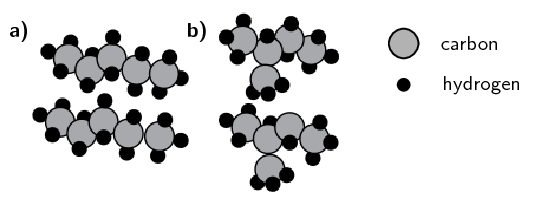(a) Straight chains of pentane and (b) branched chains of 2-methylbutane. (Image by Duncan Watson);

Shown in Figure 4.68 are three isomers that all have the molecular formula $$\text{C}_{5}\text{H}_{12}$$. They are all alkanes with the only difference being branched groups leading to longer or shorter main chains.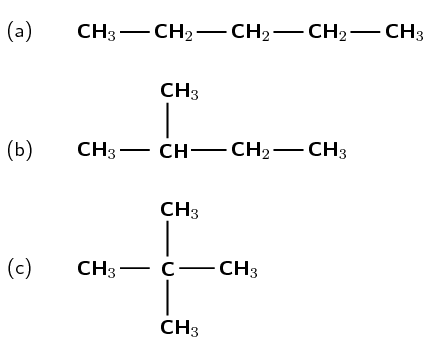Figure 4.68: Three isomers of $$\text{C}_{5}\text{H}_{12}$$: (a) pentane, (b) 2-methylbutane and (c) 2,2-dimethylpropane.

As shown in Table 4.16 the properties of these three compounds are significantly different. The melting points have significant differences and the boiling points steadily decrease with an increasing number of branched groups.

 Name Melting point (℃) Boiling point (℃) Density ($$\text{g.dm^{-3}}$$) Vapour pressure (kPa at 20℃) Flash point (℃) pentane $$-\text{130}$$ $$\text{36}$$ $$\text{621}$$ $$\text{57,9}$$ $$-\text{49}$$ 2-methyl butane $$-\text{160}$$ $$\text{27,7}$$ $$\text{616}$$ $$\text{77}$$ $$-\text{51}$$ 2,2-dimethyl propane $$-\text{16,6}$$ $$\text{9,5}$$ $$\text{586}$$ $$\text{147}$$ $$<-\text{7}$$

Table 4.16: Properties of compounds with different numbers of branched groups.

Although the densities of all three compounds are very similar, there is a decrease in density with the increase of branched groups. The vapour pressure increases with increasing branched groups and the flash point of 2,2-dimethylpropane is significantly higher than those of pentane and 2-methylbutane.

It is interesting that the common trend of melting and boiling points is not followed here - 2,2-dimethylpropane has the highest melting point, lowest boiling point and is the least flammable although all three compounds are very flammable. Symmetrical molecules (such as 2,2-dimethylpropane) tend to have higher melting points than similar molecules with less symmetry due to their packing in the solid state. Once in the liquid state they follow the normal trends.

## Physical properties and branched chains

Textbook Exercise 4.22
 Structural Formula Boiling point (℃)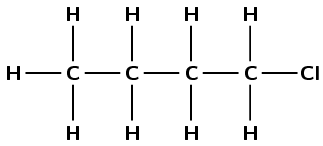$$\text{78}$$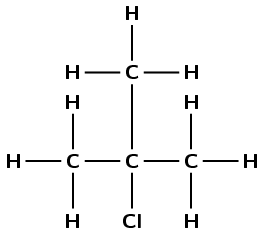$$\text{51}$$

Give the IUPAC name for each compound

1-chlorobutane and 2-chloro-2-methylpropane

Explain the difference in the melting points

The branched haloalkane (2-chloro-2-methylpropane) will have less surface area and thus weaker intermolecular forces than the straight chain haloalkane (1-chlorobutane). Less energy will be needed to break the intermolecular forces of 2-chloro-2-methylpropane, and therefore a lower boiling point is observed.

There are five isomers with the molecular formula $$\text{C}_{6}\text{H}_{14}$$.

Draw the structural representations of:

2-methylpentane and 2,2-dimethylbutane (two of the isomers)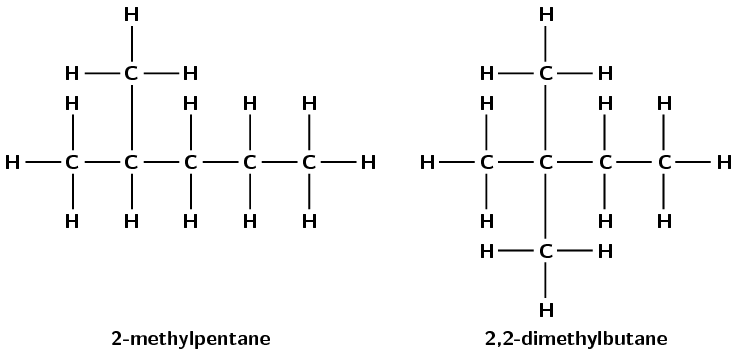What are the names of the other three isomers?

hexane, 3-methylpentane and 2,3-dimethylbutane

Draw the semi-structural representations of these three molecules.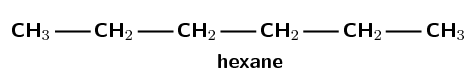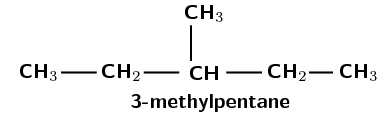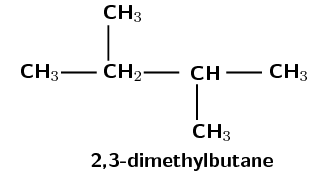The melting points of these three isomers are: $$-\text{118}$$, $$-\text{95}$$ and $$-\text{130}$$ $$\text{℃}$$. Assign the correct melting points to the correct isomer. Give a reason for your answers.

The lowest melting point will be with the molecule with the weakest intermolecular forces. This molecule is 2,3-dimethylbutane as it is the isomer with the most branched chains. The highest melting point will be with the molecule with the strongest intermolecular forces. This molecule is hexane as it is the isomer with no branched groups.

Therefore the melting points will be:

• 2,3-dimethylbutane: $$-\text{130}$$ $$\text{℃}$$

• 3-methylpentane: $$-\text{118}$$ $$\text{℃}$$

• hexane: $$-\text{95}$$ $$\text{℃}$$

## Physical properties of organic compounds

Textbook Exercise 4.23

The table shows data collected for four organic compounds (A - C) during a practical investigation.

 Compound Molecular Mass ($$\text{g.mol^{-1}}$$) Boiling point (℃) A $$\text{CH}_{3}\text{CH}_{2}\text{CH}_{3}$$ $$\text{44,08}$$ $$-\text{42}$$ B $$\text{CH}_{3}\text{CHO}$$ $$\text{44,04}$$ $$\text{20}$$ C $$\text{CH}_{3}\text{CH}_{2}\text{OH}$$ $$\text{46,06}$$ $$\text{78}$$

Is compound A saturated or unsaturated? Give a reason for your answer.

Saturated. It contains only single carbon-carbon bonds.

To which homologous series does compound B belong?

aldehydes

Write down the IUPAC name for each of the following compounds:

B

ethanal

C

ethanol

Refer to intermolecular forces to explain the difference in boiling points between compounds A and C.

Between alkane molecules/molecules of compound A/propane molecules there are only weak van der Waals forces/intermolecular forces.

Between alcohol molecules/molecules of compound D/ethanol molecules there are strong hydrogen bonds (as well as weak van der Waals forces).

More energy is needed to overcome the intermolecular forces/hydrogen bonds between alcohol molecules/ethanol molecules/molecules of compound C

OR

Less energy is needed to overcome the intermolecular forces/van der Waals forces between alkane molecules/propane molecules/molecules of compound A

Which one of compounds B or C will have the highest vapour pressure at a specific temperature? Give a reason for your answer.

B. A lower boiling point (or weaker intermolecular forces) means that more molecules will go into the vapour phase, resulting in a higher vapour pressure.

Give the IUPAC names for the following compounds and answer the questions that follow.Boiling point = $$\text{118}$$ $$\text{℃}$$

butan-1-ol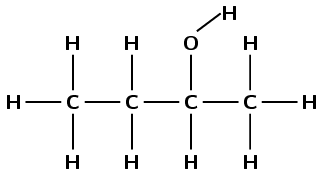Boiling point = $$\text{99}$$ $$\text{℃}$$

butan-2-ol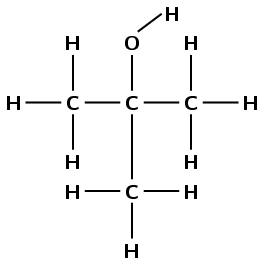Boiling point = $$\text{82,5}$$ $$\text{℃}$$

2-methylpropan-2-ol

Explain the difference in boiling points.

The hydrogen bonding hydroxyl group is located on an end carbon atom in butan-1-ol. This allows it to form hydrogen bonds more easily than butan-2-ol. The branched group in 2-methylpropan-2-ol means that it is even more difficult for hydrogen bonds to form between these molecules (weak intermolecular forces). The greater the intermolecules forces, the more energy that is required to overcome them, and the higher the boiling point will be.